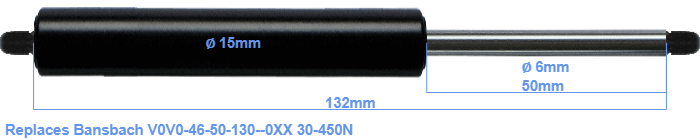# Replacement for Bansbach V0V0-46-50-130–0XX 30-450N

\$25.56

Replacement gas spring for the Bansbach V0V0-46-50-130--0XX 30-450 Newton. Thread: M5. Brand: HAHN, a Stabilus company.
 Force Choose an option30 Newton40 Newton50 Newton60 Newton80 Newton100 Newton120 Newton140 Newton150 Newton160 Newton180 Newton200 Newton220 Newton240 Newton250 Newton260 Newton280 Newton300 Newton320 Newton340 Newton350 Newton360 Newton380 Newton400 Newton420 Newton440 Newton450 NewtonClearThe diameter of the cylinder of this gas spring is 15 millimeters. The diameter of the rod totals 6 mm. The chrome part is called the rod. It has a length of 50 mm. In total, the length is 132 millimeter. This is the total distance between the centers of the two mounting parts. The distance from thread to thread (without mounting parts) is 132 mm. The force of this replacement gas spring is 30-450 Newton. Attention: this is not an official Bansbach gas spring, but a replacement gas spring. This a gas spring with the brand HAHN. Nevertheless, the dimensions and force are equal.